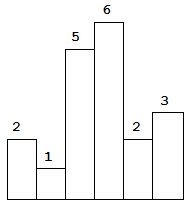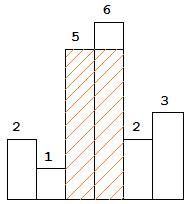## Description

Largest Rectangle in Histogram

Given n non-negative integers representing the histogram's bar height where the width of each bar is 1, find the area of largest rectangle in the histogram.Above is a histogram where width of each bar is 1, given height = [2,1,5,6,2,3].The largest rectangle is shown in the shaded area, which has area = 10 unit.

## Solution

1. 如果升序则直接入栈
2. 遇到降序，将栈中所有比h高的都利用公式计算出面积，然后降低它们的高度至h，使数组重新有序

^
|   0
|  00 0
|  0000
| 00000
.------->

^
|   0       stack:
|  00           
|  00           
| 000           
.------->

^
|           stack:  将 4 的下标出栈，保留 3 的下标（因为 3 所在位置是新高度的起点）
|
|  000          
| 0000          
.------->

^
|           stack:
|     0         
|  0000         
| 00000         
.------->


  1 2 3 4 5 6 7 8 9 10 11 12 13 14 15 16 17 18 19 20 21 22 23 24 25 26 27 28 29 30 31 32 33 34 35 36 37 38 39 40 41 42 43 44 45  func maxInt(a, b int) int { if a > b { return a } return b } func largestRectangleArea(heights []int) int { stack := make([]int, 0) lenStack := len(stack) max := 0 // 追加一个 -1，用于最后清空栈 heights = append(heights, -1) for i := 0; i < len(heights); i++ { h := heights[i] if lenStack > 0 && h < heights[stack[lenStack-1]] { // 如果前面更高，将更高的都出栈 // 同时计算面积，然后降低它们的高度（形成新的“有序”） for { idx := stack[lenStack-1] // 计算面积 max = maxInt(max, heights[idx]*(i-idx)) // 降低高度 heights[idx] = h lenStack-- // 假出栈 if lenStack == 0 || h > heights[stack[lenStack-1]] { // 最后一个，保留在栈中作为新高度的起点 lenStack++ break } stack = stack[:lenStack] } } else /* 这个判断可有可无 if lenStack == 0 || h > heights[stack[lenStack-1]] */ { // 将当前索引入栈 stack = append(stack, i) lenStack++ } } return max }